## Example Questions

### Example Question #1491 : Act Math

A wedding planner knows she must deliver 85 small fruits and 40 large fruits to the wedding event. She knows that one basket can hold either 5 small fruits or 3 large fruits. What is the minimum number of baskets she must bring to the event to fulfill their demand?Explanation:

To know the minumum number of baskets required for each size, simply divide the number of fruits required by the basket capacity for each fruit: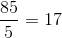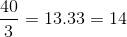(we must round up to nearest whole number for practical purposes)

Since we need to bring both the large and small fruits to the event, we need to add these two values: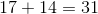baskets total.

### Example Question #109 : Fractions

After saving her money for six weeks, Felicia has saved up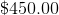. If she saves three-fifths of her pay each week, how much does she make in a week?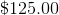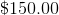Cannot be determined from the information provided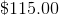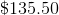Explanation:

When working with fractions or percents, it is often really helpful to write out an equation showing what you need to find. In this case, we know that three-fifths of Felicia's weekly pay (our variable—let's call it) over six weeks results in, so our equation looks like this: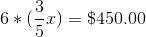So, we want to simplify and rearrange this equation. Start by multiplying thewith the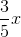: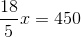Then, multiply both sides by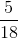, the reciprocal of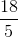: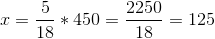So,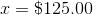, and we now know Felicia's weekly pay!

### Example Question #110 : Fractions

A company needs 45 pounds of apples to make 30 containers of apple juice. How many pounds of apples would the company need to make 20 containers of apple juice?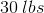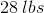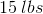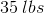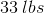Explanation:

To answer this question, we need to set up a proportion for how many pounds of apples it takes to make a certain number of containers, knowing that 45 pounds of apples makes 30 containers of apple juice. We want to figure out how many pounds of apples it would take to make 20 containers of apple juice.

To set up a proportion, we must put the amounts that correspond to each other in the same fraction and equate it to a similar fraction. In this case, we will set up fractions that show containers of apple juice over pounds of apples. So for this data: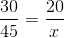Note that we put both of the values for containers of apple juice in the numerator of the fractions and pounds of apples in the denominator. Here,is the value we are looking for.

We then cross-multiply by taking the denominator of each fraction and multiplying each side of the equation by it so that we can create an equation that is solved easier.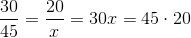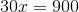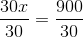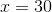Therefore, it takespounds of apples to makecontainers of apple juice.